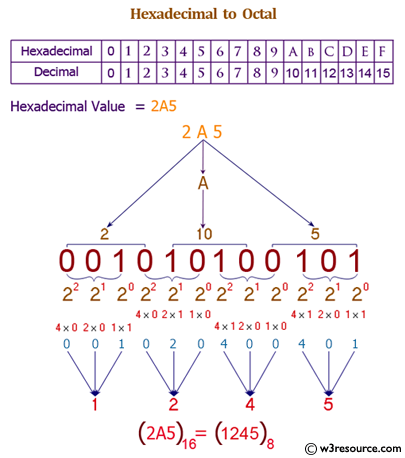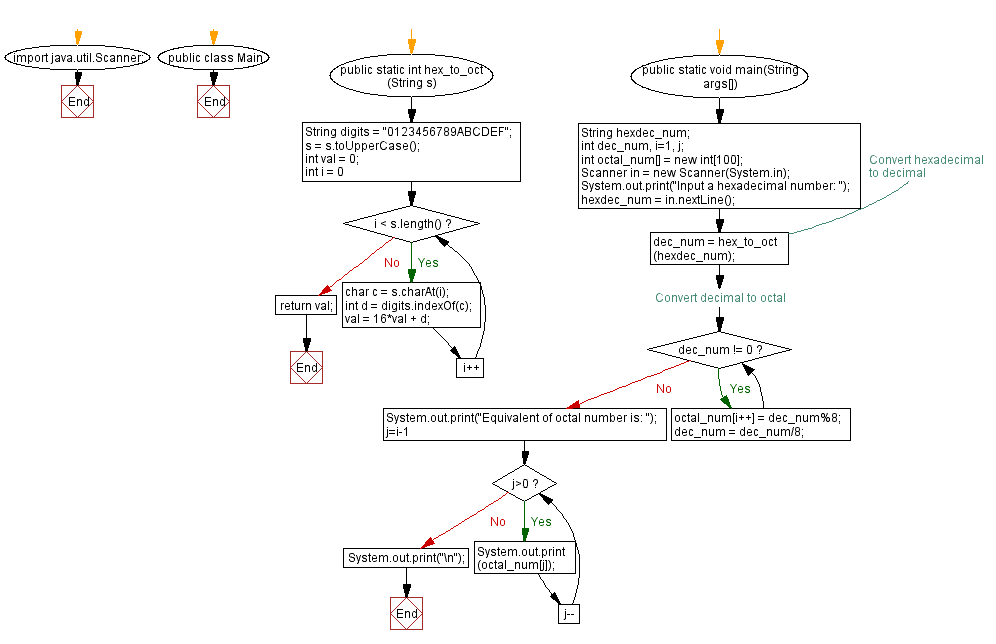﻿ Java exercises: Convert a hexadecimal to a octal number - w3resource# Java Exercises: Convert a hexadecimal to a octal number

## Java Basic: Exercise-30 with Solution

Write a Java program to convert a hexadecimal to a octal number.

Hexadecimal number: This is a positional numeral system with a radix, or base, of 16. Hexadecimal uses sixteen distinct symbols, most often the symbols 0-9 to represent values zero to nine, and A, B, C, D, E, F (or alternatively a, b, c, d, e, f) to represent values ten to fifteen.

Octal number: The octal numeral system is the base-8 number system, and uses the digits 0 to 7.

Test Data:

Pictorial Presentation: Hexadecimal to Octal numberSample Solution:

Java Code:

``````import java.util.Scanner;

public class Exercise30 {
public static int hex_to_decimal(String s)
{
String digits = "0123456789ABCDEF";
s = s.toUpperCase();
int val = 0;
for (int i = 0; i < s.length(); i++)
{
char c = s.charAt(i);
int d = digits.indexOf(c);
val = 16*val + d;
}
return val;
}
public static void main(String args[])
{
String hexdec_num;
int dec_num, i=1, j;
int octal_num[] = new int;
Scanner in = new Scanner(System.in);

hexdec_num = in.nextLine();

dec_num = hex_to_decimal(hexdec_num);

//Convert decimal to octal

while(dec_num != 0)
{
octal_num[i++] = dec_num%8;
dec_num = dec_num/8;
}

System.out.print("Equivalent of octal number is: ");
for(j=i-1; j>0; j--)
{
System.out.print(octal_num[j]);
}
System.out.print("\n");
}
}
```
```

Sample Output:

```Input a hexadecimal number: 40
Equivalent of octal number is: 100
```

Flowchart:Java Code Editor:

What is the difficulty level of this exercise?

Test your Programming skills with w3resource's quiz.

﻿

## Java: Tips of the Day

getEnumMap

Converts to enum to Map where key is the name and value is Enum itself.

```public static <E extends Enum<E>> Map<String, E> getEnumMap(final Class<E> enumClass) {
return Arrays.stream(enumClass.getEnumConstants())
.collect(Collectors.toMap(Enum::name, Function.identity()));
}
```

Ref: https://bit.ly/3xXcFZt